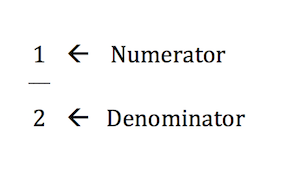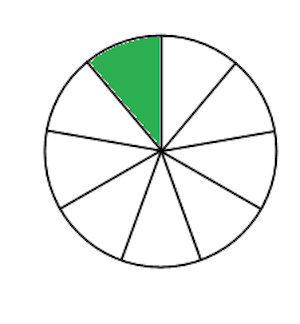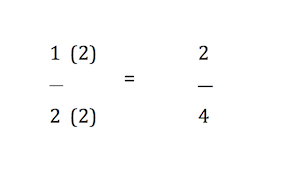# How to Subtract Fractions with Unlike Denominators

Instructor: Julie Zundel

Julie has taught high school Zoology, Biology, Physical Science and Chem Tech. She has a Bachelor of Science in Biology and a Master of Education.

Subtracting fractions is fairly straightforward, if you know the rules. This lesson will give you the steps needed to subtract fractions and then will go over additional examples.

## How to Solve

This lesson explores how to subtract fractions with unlike denominators. A fraction is a number that isn't a whole, and it is broken up into a numerator, or the top number, and a denominator, or bottom number.The denominator depicts how many equal pieces make up the whole and the numerator depicts how many of those pieces you have. For example, if you have a pie and cut it into 9 pieces, the denominator is 9. If you eat one of those pieces, the numerator is 1. So you ate 1/9 of a pie.Let's go over the steps needed to solve these sorts of problems:

• Step 1: Make the denominators are the same.
• Find the common denominator. This is a number both denominators can go into.
• Step 2: Rewrite the fractions using the new denominators.
• In order to change the denominators, you must multiply the numerator and the denominator by the number it took to change the denominator.
• Step 3: Subtract the numerators
• The last step is subtracting the numerators and leaving the denominator the same.

Now that you're familiar with the steps, let's see it in action with an actual problem: 1/2-1/4. Let's follow the steps we went over earlier.

• Step 1: Make the denominators are the same.
• This means you will make both 1/2 and 1/4 have the same bottom number. This must be a number that BOTH denominators, or 2 and 4, can be divided into. And here we will want to use the least common denominator, meaning the smallest number 2 and 4 can both be divided into. Here the least common denominator is 4 because 2 can go into 4 and 4 can go into 4.
• Step 2: Rewrite the fractions using the new denominators.
• In order to get the denominator of 1/2 to a 4, you would multiply by 2, so you need to multiply the numerator AND the denominator by 2, as illustrated. Next, look at 1/4. The denominator is already 4, so you do not have to multiply it by anything.To unlock this lesson you must be a Study.com Member.

### Register to view this lesson

Are you a student or a teacher?

#### See for yourself why 30 million people use Study.com

##### Become a Study.com member and start learning now.
Back
What teachers are saying about Study.com

### Earning College Credit

Did you know… We have over 200 college courses that prepare you to earn credit by exam that is accepted by over 1,500 colleges and universities. You can test out of the first two years of college and save thousands off your degree. Anyone can earn credit-by-exam regardless of age or education level.